A particle is given a constant horizontal velocity  from height h. Taking g to be constant every where, kinetic energy E of the particle w. r. t. time t is correctly shown in

(1)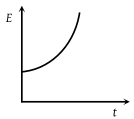(2)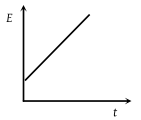(3)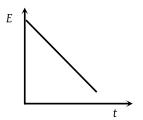(4)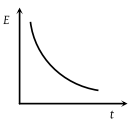Concept Questions :-

Concept of work
High Yielding Test Series + Question Bank - NEET 2020

Difficulty Level:

The adjoining diagram shows the velocity versus time plot for a particle. The work done by the force on the particle is positive from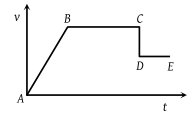(1) A to B

(2) B to C

(3) C to D

(4) D to E

Concept Questions :-

Concept of work
High Yielding Test Series + Question Bank - NEET 2020

Difficulty Level:

A particle which is constrained to move along the x-axis, is subjected to a force in the same direction which varies with the distance x of the particle from the origin as $F\left(x\right)=-kx+a{x}^{3}$. Here k and a are positive constants. For $x\ge 0$, the functional from of the potential energy U(x) of the particle is-

(1)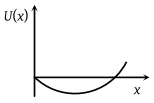(2)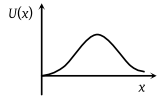(3)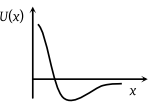(4)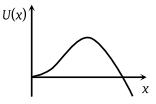Concept Questions :-

Potential energy: Relation with force
High Yielding Test Series + Question Bank - NEET 2020

Difficulty Level:

A force F acting on an object varies with distance x as shown here. The force is in newton and x in metre. The work done by the force in moving the object from x = 0 to x = 6 m is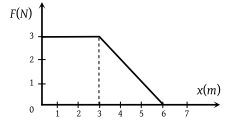(1) 4.5 J

(2) 13.5 J

(3) 9.0 J

(4) 18.0 J

Concept Questions :-

Work done by variable force
High Yielding Test Series + Question Bank - NEET 2020

Difficulty Level:

The potential energy of a system is represented in the first figure. the force acting on the system will be represented by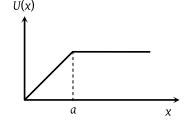(1)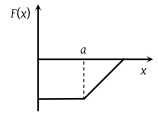(2)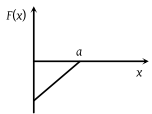(3)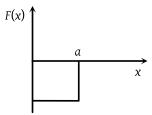(4)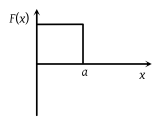Concept Questions :-

Potential energy: Relation with force
High Yielding Test Series + Question Bank - NEET 2020

Difficulty Level:

A particle, initially at rest on a frictionless horizontal surface, is acted upon by a horizontal force which is constant in size and direction. A graph is plotted between the work done (W) on the particle, against the speed of the particle, (v). If there are no other horizontal forces acting on the particle the graph would look like

(1)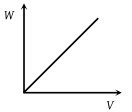(2)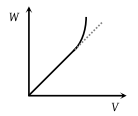(3)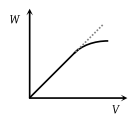(4)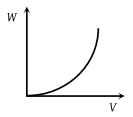Concept Questions :-

Work-Energy theorem
High Yielding Test Series + Question Bank - NEET 2020

Difficulty Level:

Which of the following graphs is correct between kinetic energy (E), potential energy (U) and height (h) from the ground of the particle

(1)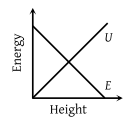(2)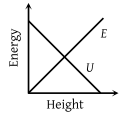(3)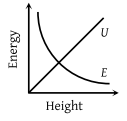(4)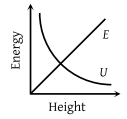Concept Questions :-

Gravitational Potential energy
High Yielding Test Series + Question Bank - NEET 2020

Difficulty Level:

The graph between $\sqrt{E}$ and $\frac{1}{p}$ is (E = kinetic energy and p = momentum)

1.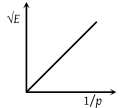2.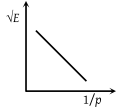3.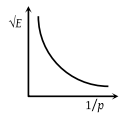4.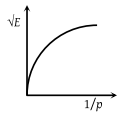Concept Questions :-

Concept of work
High Yielding Test Series + Question Bank - NEET 2020

Difficulty Level:

The force acting on a body moving along x-axis varies with the position of the particle as shown in the fig.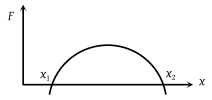The body is in stable equilibrium at

(1) x = x1

(2) x = x2

(3) both x1 and x2

(4) neither x1 nor x2

Concept Questions :-

Potential energy: Relation with force
High Yielding Test Series + Question Bank - NEET 2020

Difficulty Level:

The potential energy of a particle varies with distance x as shown in the graph. The force acting on the particle is zero at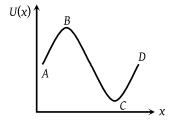(1) C

(2) B

(3) B and C

(4) A and D

Concept Questions :-

Potential energy: Relation with force